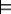#### 9.3.2.1 Expected Utility of a Policy

A policy can be evaluated by determining its expected utility for an agent following the policy. A rational agent should adopt the policy that maximizes its expected utility.

A possible world specifies a value for each random variable and each decision variable. A possible world does not have a probability unless values for all of the decision variables are specified. A possible world satisfies a policy if the value of each decision variable in the possible world is the value selected in the decision function for that decision variable in the policy. If ω is a possible world, and π is a policy, ωπ is defined to mean that possible world ω satisfies policy π.

It is important to realize that a possible world corresponds to a complete history and specifies the values of all random and decision variables, including all observed variables, for a complete sequence of actions. Possible world ω satisfies policy π if ω is one possible unfolding of history given that the agent follows policy π. The satisfiability constraint enforces the intuition that the agent will actually do the action prescribed by π for each of the possible observations.

The expected utility of policy π is

E(π) = ∑ωπ U(ω) ×P(ω),

where P(ω), the probability of world ω, is the product of the probabilities of the values of the chance nodes given their parents' values in ω, and U(ω) is the value of the utility U in world ω.

Example 9.16: In Example 9.11, let π1 be the policy to take the umbrella if the forecast is cloudy and to leave it at home otherwise. The expected utility of this policy is obtained by averaging the utility over the worlds that satisfy this policy:
 E(π1) = P(norain) P(sunny|norain) Utility(norain,leaveIt) + P(norain) P(cloudy|norain) Utility(norain,takeIt) + P(norain) P(rainy|norain) Utility(norain,leaveIt) + P(rain) P(sunny|rain) Utility(rain,leaveIt) + P(rain) P(cloudy|rain) Utility(rain,takeIt) + P(rain) P(rainy|rain) Utility(rain,leaveIt),

where norain means Weather=norain, sunny means Forecast=sunny, and similarly for the other values. Notice how the value for the decision variable is the one chosen by the policy. It only depends on the forecast.

An optimal policy is a policy π* such that E*) ≥ E(π) for all policies E(π). That is, an optimal policy is a policy whose expected utility is maximal over all policies.

Suppose a binary decision node has n binary parents. There are 2n different assignments of values to the parents and, consequently, there are 22n different possible decision functions for this decision node. The number of policies is the product of the number of decision functions for each of the decision variables. Even small examples can have a huge number of policies. Thus, an algorithm that enumerates the set of policies looking for the best one will be very inefficient.